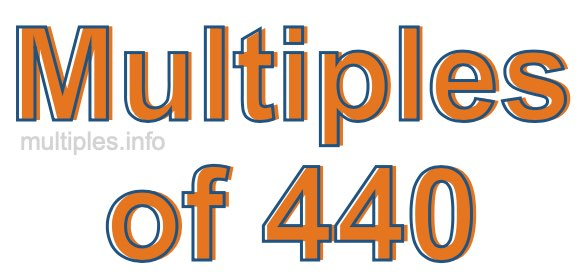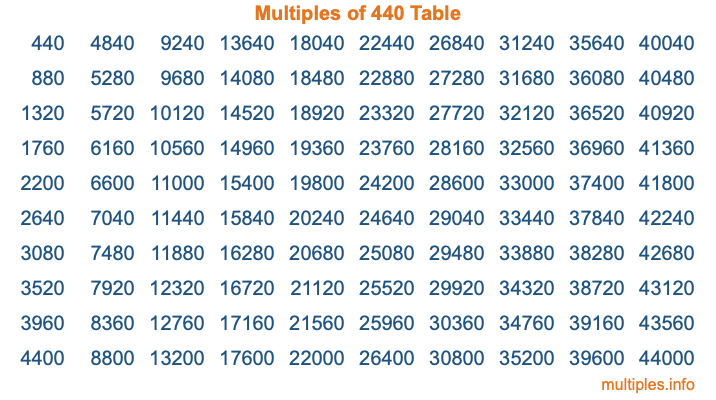Multiples of 440Welcome to the Multiples of 440 page. Here we will first teach you everything you will ever need to know about the multiples of 440, and then give you a study guide summary of everything we taught you to make sure you remember it all. Use this page to look up facts and learn information about the multiples of 440. This page will make you a multiples of four hundred forty expert!

Definition of Multiples of 440
Multiples of 440 are all the numbers that when divided by 440 equal an integer. Each of the multiples of 440 are called a multiple. A multiple of 440 is created by multiplying 440 by an integer.

Therefore, to create a list of multiples of 440, you start with 1 multiplied by 440, then 2 multiplied by 440, then 3 multiplied by 440, and so on for as long as you want. Thus, the list of the first five multiples of 440 is 440, 880, 1320, 1760, and 2200. To see a larger list of multiples of 440, see the printable image of Multiples of 440 further down on this page. We also have a category where you can choose any nth multiple of 440.

Multiples of 440 Checker
The Multiples of 440 Checker below checks to see if any number of your choice is a multiple of 440. In other words, it checks to see if there is any number (integer) that when multiplied by 440 will equal your number. To do that, we divide your number by 440. If the the quotient is an integer, then your number is a multiple of 440.

Is  a multiple of 440?

Least Common Multiple of 440 and ...
A Least Common Multiple (LCM) is the lowest multiple that two or more numbers have in common. This is also called the smallest common multiple or lowest common multiple and is useful to know when you are adding our subtracting fractions. Enter one or more numbers below (440 is already entered) to find the LCM.

Check out our LCM Calculator if you need more details about the Least Common Multiple or if you need the LCM for different numbers for adding and subtraction fractions.

nth Multiple of 440
As we stated above, 440 is the first multiple of 440, 880 is the second multiple of 440, 1320 is the third multiple of 440, and so on. Enter a number below to find the nth multiple of 440.

th multiple of 440

Multiples of 440 vs Factors of 440
440 is a multiple of 440 and a factor of 440, but that is where the similarities end. All postive multiples of 440 are 440 or greater than 440. All positive factors of 440 are 440 or less than 440.

Below is the beginning list of multiples of 440 and the factors of 440 so you can compare:

Multiples of 440: 440, 880, 1320, 1760, 2200, etc.

Factors of 440: 1, 2, 4, 5, 8, 10, 11, 20, 22, 40, 44, 55, 88, 110, 220, 440

As you can see, the multiples of 440 are all the numbers that you can divide by 440 to get a whole number. The factors of 440, on the other hand, are all the whole numbers that you can multiply by another whole number to get 440.

It's also interesting to note that if a number (x) is a factor of 440, then 440 will also be a multiple of that number (x).

Multiples of 440 vs Divisors of 440
The divisors of 440 are all the integers that 440 can be divided by evenly. Below is a list of the divisors of 440.

Divisors of 440: 1, 2, 4, 5, 8, 10, 11, 20, 22, 40, 44, 55, 88, 110, 220, 440

The interesting thing to note here is that if you take any multiple of 440 and divide it by a divisor of 440, you will see that the quotient is an integer.

Multiples of 440 Table
Below is an image of the first 100 multiples of 440 in a table. The table is in chronological order, column by column. The first column has the first ten multiples of 440, the second column has the next ten multiples of 440, and so on.The Multiples of 440 Table is also referred to as the 440 Times Table or Times Table of 440. You are welcome to print out our table for your studies.

Negative Multiples of 440
Although not often discussed or needed in math, it is worth mentioning that you can make a list of negative multiples of 440 by multiplying 440 by -1, then by -2, then by -3, and so on, to get the following list of negative multiples of 440:

-440, -880, -1320, -1760, -2200, etc.

Multiples of 440 Summary
Below is a summary of important Multiples of 440 facts that we have discussed on this page. To retain the knowledge on this page, we recommend that you read through the summary and explain to yourself or a study partner why they hold true.

There are an infinite number of multiples of 440.

A multiple of 440 divided by 440 will equal a whole number.

440 divided by a factor of 440 equals a divisor of 440.

The nth multiple of 440 is n times 440.

The largest factor of 440 is equal to the first positive multiple of 440.

440 is a multiple of every factor of 440.

440 is a multiple of 440.

A multiple of 440 divided by a divisor of 440 equals an integer.

440 divided by a divisor of 440 equals a factor of 440.

Any integer times 440 will equal a multiple of 440.

Multiples of a Number
Here you can get the multiples of another number, all with the same attention to detail as we did for multiples of 440 on this page.

Multiples of
Multiples of 441
Did you find our page about multiples of four hundred forty educational? Do you want more knowledge? Check out the multiples of the next number on our list!